# 10 种横盘交易策略的比较分析

17 八月 2018, 09:37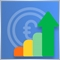0
13 504

### 在创建横盘交易策略时定义任务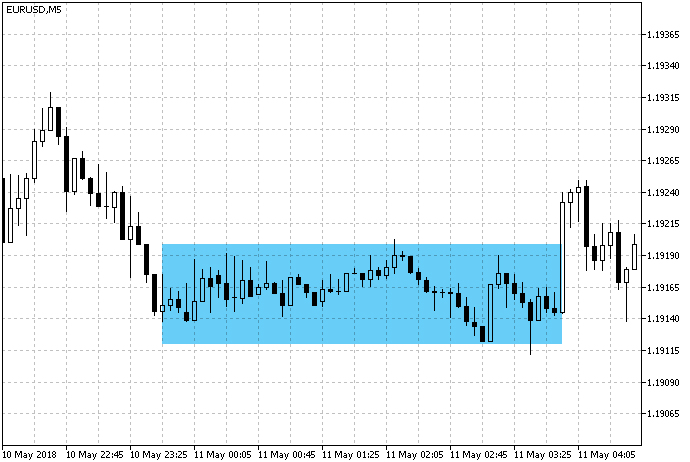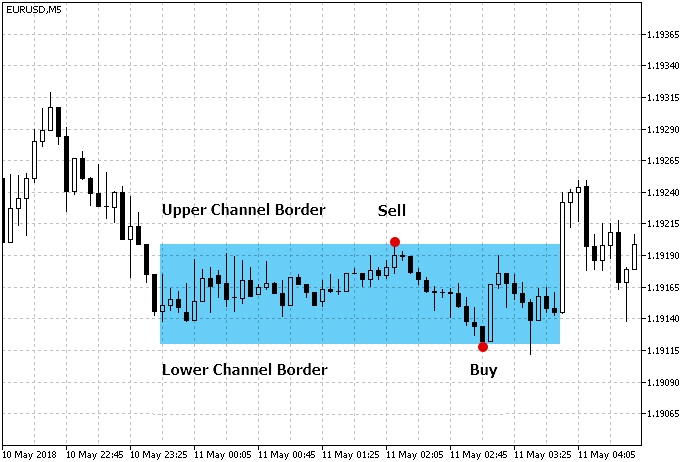### 横盘交易策略

• 交易将在通道内进行。 因此，我们需要选择能够帮助我们构建通道，并确定横盘区域虚拟边界的工具。
• 除了定义通道之外，我们还需要至少一个额外的工具来确认价格在从通道边界反弹后将朝着正确的方向发展。 这种滤波器的目的就是避免入场假信号。

#### 策略 #1，附带基于 MFI 滤波器的轨道线指标

通道边界基于轨道线指标判断。 MFI 指标还用于过滤信号。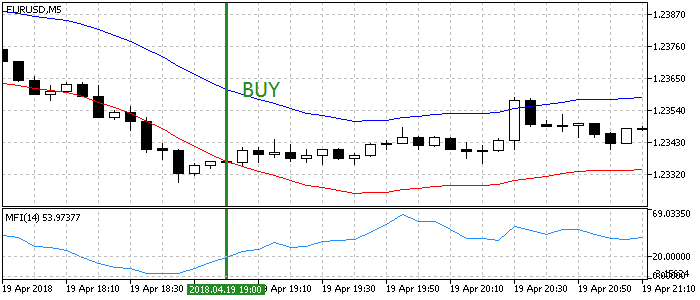```void OnTick()
{
//--- 检查以前由该 EA 开立的订单
{
//--- 获取数据进行计算
if(!GetIndValue())
return;
//--- 如果有买入信号，则开单

//--- 如果有卖出信号，则开单
if(SellSignal())
}
}
//+------------------------------------------------------------------+
//| 买入条件                                                          |
//+------------------------------------------------------------------+
{
if(mfi<20 && env_low>close)
{
tp=env_high;
return true;
}
else
return false;
}
//+------------------------------------------------------------------+
//| 卖出条件                                                          |
//+------------------------------------------------------------------+
bool SellSignal()
{
if(mfi>80 && env_high<close)
{
tp=env_low;
return true;
}
else
return false;
}
//+------------------------------------------------------------------+
//| 获取指标的当前值                                                  |
//+------------------------------------------------------------------+
bool GetIndValue()
{
return(CopyBuffer(InpInd_Handle1,0,0,2,mfi)<=0  ||
CopyBuffer(InpInd_Handle2,1,0,2,env_low)<=0 ||
CopyBuffer(InpInd_Handle2,0,0,2,env_high)<=0 ||
CopyClose(Symbol(),PERIOD_CURRENT,0,2,close)<=0
)?false:true;
}
//+------------------------------------------------------------------+
```

#### 策略 #2，布林带加两条移动均线```void OnTick()
{
//--- 检查以前由该 EA 开立的订单
{
//--- 获取数据进行计算
if(!GetIndValue())
return;
//--- 如果有买入信号，则开单

//--- 如果有卖出信号，则开单
if(SellSignal())
}
}
//+------------------------------------------------------------------+
//| 买入条件                                                          |
//+------------------------------------------------------------------+
{
if(ma_slow>ma_fast && bb_low>close)
{
tp=bb_up;
return true;
}
else
return false;
}
//+------------------------------------------------------------------+
//| 卖出条件                                                          |
//+------------------------------------------------------------------+
bool SellSignal()
{
if(ma_slow<ma_fast && bb_up<close)
{
tp=bb_low;
return true;
}
else
return false;
}
//+------------------------------------------------------------------+
//| 获取指标的当前值                                                    |
//+------------------------------------------------------------------+
bool GetIndValue()
{
return(CopyBuffer(InpInd_Handle1,1,0,2,bb_up)<=0  ||
CopyBuffer(InpInd_Handle1,2,0,2,bb_low)<=0 ||
CopyBuffer(InpInd_Handle2,0,0,2,ma_slow)<=0 ||
CopyBuffer(InpInd_Handle3,0,0,2,ma_fast)<=0 ||
CopyClose(Symbol(),PERIOD_CURRENT,0,2,close)<=0
)?false:true;
}
//+------------------------------------------------------------------+
```

#### 策略 #3. WSO & WRO 通道和埃勒斯 (Ehlers) 分形维度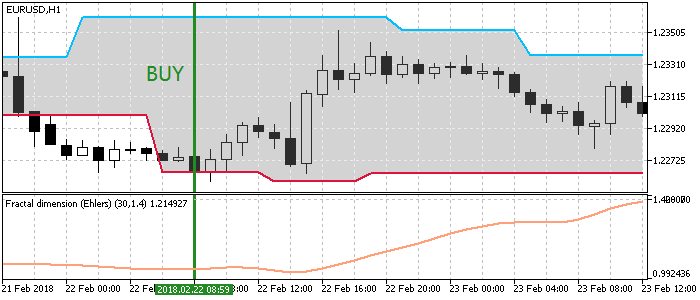```void OnTick()
{
//--- 检查以前由该 EA 开立的订单
{
//--- 获取数据进行计算
if(!GetIndValue())
return;
//--- 如果有买入信号，则开单

//--- 如果有卖出信号，则开单
if(SellSignal())
}
}
//+------------------------------------------------------------------+
//| 买入条件                                                          |
//+------------------------------------------------------------------+
{
if(wwc_low>close && fdi<Inp_FdiThreshold)
{
tp=wwc_up;
return true;
}
else
return false;
}
//+------------------------------------------------------------------+
//| 卖出条件                                                          |
//+------------------------------------------------------------------+
bool SellSignal()
{
if(wwc_up<close && fdi<Inp_FdiThreshold)
{
tp=wwc_low;
return true;
}
else
return false;
}
//+------------------------------------------------------------------+
//| 获取指标的当前值                                                    |
//+------------------------------------------------------------------+
bool GetIndValue()
{
return(CopyBuffer(InpInd_Handle1,3,0,2,wwc_up)<=0  ||
CopyBuffer(InpInd_Handle1,2,0,2,wwc_low)<=0 ||
CopyBuffer(InpInd_Handle2,0,0,2,fdi)<=0 ||
CopyClose(Symbol(),PERIOD_CURRENT,0,2,close)<=0
)?false:true;
}
//+------------------------------------------------------------------+
```

#### 策略 #4， 通道交叉百分比指标并基于趋势范围滤波器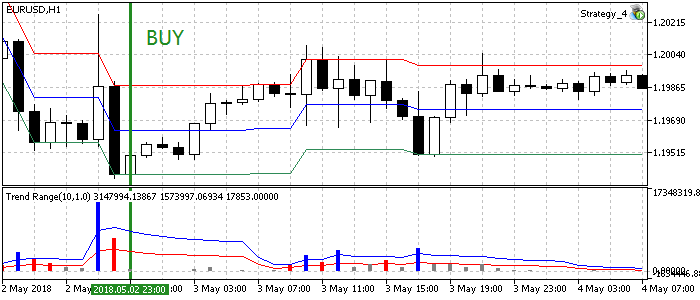```void OnTick()
{
//--- 检查以前由该 EA 开立的订单
{
//--- 获取数据进行计算
if(!GetIndValue())
return;
//--- 如果有买入信号，则开单

//--- 如果有卖出信号，则开单
if(SellSignal())
}
}
//+------------------------------------------------------------------+
//| 买入条件                                                          |
//+------------------------------------------------------------------+
{
if(pcc_low>close && tr_flat>tr_range)
{
tp=pcc_up;
return true;
}
else
return false;
}
//+------------------------------------------------------------------+
//| 卖出条件                                                          |
//+------------------------------------------------------------------+
bool SellSignal()
{
if(pcc_up<close && tr_flat>tr_range)
{
tp=pcc_low;
return true;
}
else
return false;
}
//+------------------------------------------------------------------+
//| 获取指标的当前值                                                    |
//+------------------------------------------------------------------+
bool GetIndValue()
{
return(CopyBuffer(InpInd_Handle1,0,0,2,pcc_up)<=0  ||
CopyBuffer(InpInd_Handle1,2,0,2,pcc_low)<=0 ||
CopyBuffer(InpInd_Handle2,1,0,2,tr_flat)<=0 ||
CopyBuffer(InpInd_Handle2,2,0,2,tr_range)<=0 ||
CopyClose(Symbol(),PERIOD_CURRENT,0,2,close)<=0
)?false:true;
}
//+------------------------------------------------------------------+
```

#### 策略 #5， 价格通道指标和基于 RBVI 的滤波器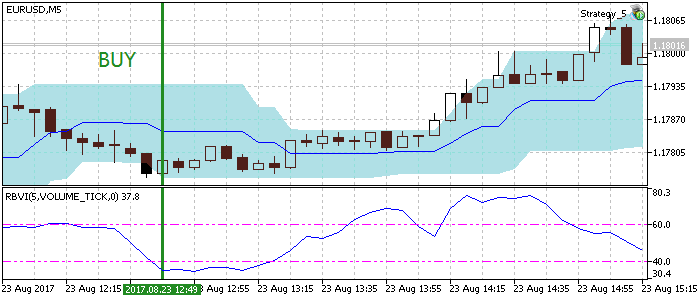```void OnTick()
{
//--- 检查以前由该 EA 开立的订单
{
//--- 获取数据进行计算
if(!GetIndValue())
return;

//--- 如果有买入信号，则开单

//--- 如果有卖出信号，则开单
if(SellSignal())
}
}
//+------------------------------------------------------------------+
//| 买入条件                                                          |
//+------------------------------------------------------------------+
{
if(pc_low>close && rbvi<=Inp_level)
{
tp=pc_up;
return true;
}
else
return false;
}
//+------------------------------------------------------------------+
//| 卖出条件                                                          |
//+------------------------------------------------------------------+
bool SellSignal()
{
if(pc_up<close && rbvi<=Inp_level)
{
tp=pc_low;
return true;
}
else
return false;
}
//+------------------------------------------------------------------+
//| 获取指标的当前值                                                    |
//+------------------------------------------------------------------+
bool GetIndValue()
{
return(CopyBuffer(InpInd_Handle1,0,0,2,pc_up)<=0  ||
CopyBuffer(InpInd_Handle1,1,0,2,pc_low)<=0 ||
CopyBuffer(InpInd_Handle2,0,0,2,rbvi)<=0 ||
CopyClose(Symbol(),PERIOD_CURRENT,0,2,close)<=0
)?false:true;
}
//+------------------------------------------------------------------+
```

#### 策略 #6， 威廉姆斯百分比范围指标和基于 ADX 的滤波器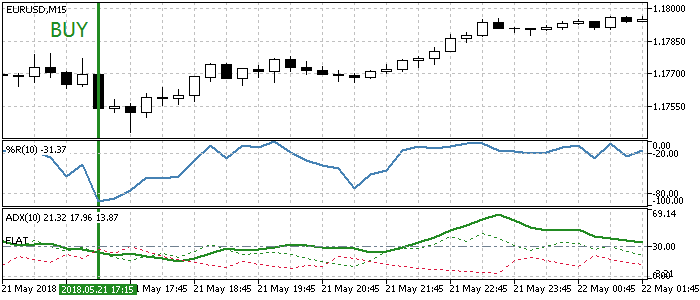```void OnTick()
{
//--- 检查以前由该 EA 开立的订单
{
//--- 获取数据进行计算
if(!GetIndValue())
return;

//--- 如果有买入信号，则开单

//--- 如果有卖出信号，则开单
if(SellSignal())
}
}
//+------------------------------------------------------------------+
//| 买入条件                                                          |
//+------------------------------------------------------------------+
{
}
//+------------------------------------------------------------------+
//| 卖出条件                                                          |
//+------------------------------------------------------------------+
bool SellSignal()
{
}
//+------------------------------------------------------------------+
//| 获取指标的当前值                                                    |
//+------------------------------------------------------------------+
bool GetIndValue()
{
return(CopyBuffer(InpInd_Handle1,0,0,2,wpr)<=0  ||
}
//+------------------------------------------------------------------+
```

#### 策略 #7， 改编的 Keltner 通道和基于魔幻趋势的滤波器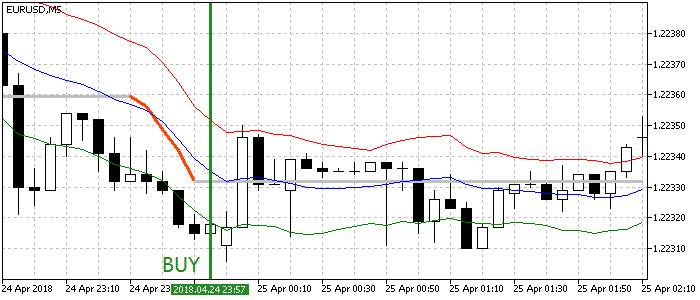```void OnTick()
{
//--- 检查以前由该 EA 开立的订单
{
//--- 获取数据进行计算
if(!GetIndValue())
return;

//--- 如果有买入信号，则开单

//--- 如果有卖出信号，则开单
if(SellSignal())
}
}
//+------------------------------------------------------------------+
//| 买入条件                                                          |
//+------------------------------------------------------------------+
{
if(kc_low>close && mt==mt)
{
tp=kc_up;
return true;
}
else
return false;
}
//+------------------------------------------------------------------+
//| 卖出条件                                                          |
//+------------------------------------------------------------------+
bool SellSignal()
{
if(kc_up<close && mt==mt)
{
tp=kc_low;
return true;
}
else
return false;
}
//+------------------------------------------------------------------+
//| 获取指标的当前值                                                    |
//+------------------------------------------------------------------+
bool GetIndValue()
{
return(CopyBuffer(InpInd_Handle1,0,0,2,kc_up)<=0  ||
CopyBuffer(InpInd_Handle1,2,0,2,kc_low)<=0 ||
CopyBuffer(InpInd_Handle2,0,0,2,mt)<=0 ||
CopyClose(Symbol(),PERIOD_CURRENT,0,2,close)<=0
)?false:true;
}
//+------------------------------------------------------------------+
```

#### 策略 #8， 唐奇安 (Donchian) 通道，由三位一体脉动 (Trinity Impulse) 确认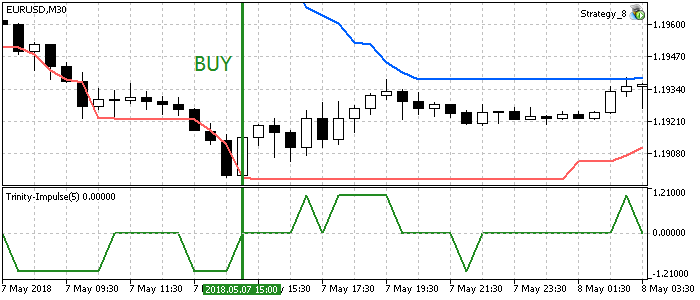```void OnTick()
{
//--- 检查以前由该 EA 开立的订单
{
//--- 获取数据进行计算
if(!GetIndValue())
return;

//--- 如果有买入信号，则开单

//--- 如果有卖出信号，则开单
if(SellSignal())
}
}
//+------------------------------------------------------------------+
//| 买入条件                                                          |
//+------------------------------------------------------------------+
{
if(dc_low>close && ti==0)
{
tp=dc_up;
return true;
}
else
return false;
}
//+------------------------------------------------------------------+
//| 卖出条件                                                          |
//+------------------------------------------------------------------+
bool SellSignal()
{
if(dc_up<close && ti==0)
{
tp=dc_low;
return true;
}
else
return false;
}
//+------------------------------------------------------------------+
//| 获取指标的当前值                                                    |
//+------------------------------------------------------------------+
bool GetIndValue()
{
return(CopyBuffer(InpInd_Handle1,0,0,2,dc_up)<=0  ||
CopyBuffer(InpInd_Handle1,1,0,2,dc_low)<=0 ||
CopyBuffer(InpInd_Handle2,0,0,2,ti)<=0 ||
CopyClose(Symbol(),PERIOD_CURRENT,0,2,close)<=0
)?false:true;
}
//+------------------------------------------------------------------+
```

#### 策略 #9， ATR 通道指标和基于 CCI 色阶的滤波器

ATR 通道基于 ATR 与移动平均线的偏差。 CCI 色阶是一个 CCI 指标，显示为阈值的直方图，表示价格变动。 我们使用此指标来过滤通道的横盘状态 (当 CCI 介于阈值之间时)。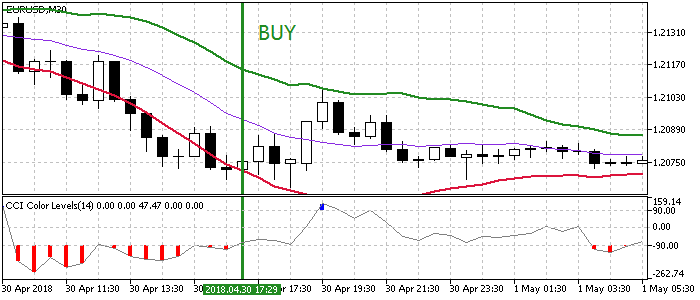```void OnTick()
{
//--- 检查以前由该 EA 开立的订单
{
//--- 获取数据进行计算
if(!GetIndValue())
return;

//--- 如果有买入信号，则开单

//--- 如果有卖出信号，则开单
if(SellSignal())
}
}
//+------------------------------------------------------------------+
//| 买入条件                                                          |
//+------------------------------------------------------------------+
{
if(atr_low>close && cci<Inp_CCI_LevelUP && cci>Inp_CCI_LevelDOWN)
{
tp=atr_up;
return true;
}
else
return false;
}
//+------------------------------------------------------------------+
//| 卖出条件                                                          |
//+------------------------------------------------------------------+
bool SellSignal()
{
if(atr_up<close && cci<Inp_CCI_LevelUP && cci>Inp_CCI_LevelDOWN)
{
tp=atr_low;
return true;
}
else
return false;
}
//+------------------------------------------------------------------+
//| 获取指标的当前值                                                    |
//+------------------------------------------------------------------+
bool GetIndValue()
{
return(CopyBuffer(InpInd_Handle1,1,0,2,atr_up)<=0  ||
CopyBuffer(InpInd_Handle1,2,0,2,atr_low)<=0 ||
CopyBuffer(InpInd_Handle2,2,0,2,cci)<=0 ||
CopyClose(Symbol(),PERIOD_CURRENT,0,2,close)<=0
)?false:true;
}
//+------------------------------------------------------------------+
```

#### 策略 #10， RSI 直方图和基于横盘指标的滤波器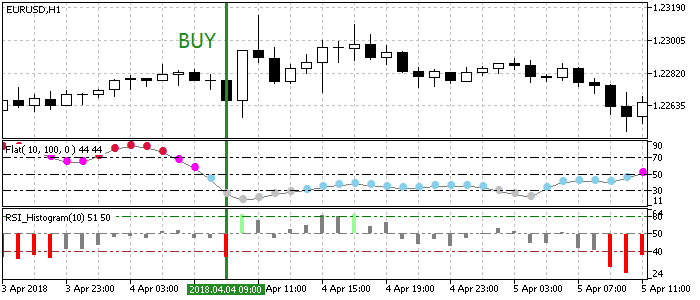```void OnTick()
{
//--- 检查以前由该 EA 开立的订单
{
//--- 获取数据进行计算
if(!GetIndValue())
return;

//--- 如果有买入信号，则开单

//--- 如果有卖出信号，则开单
if(SellSignal())
}
}
//+------------------------------------------------------------------+
//| 买入条件                                                          |
//+------------------------------------------------------------------+
{
return(rsi<Inp_LowLevel && fl<Inp_FLowLevel)?true:false;
}
//+------------------------------------------------------------------+
//| 卖出条件                                                          |
//+------------------------------------------------------------------+
bool SellSignal()
{
return(rsi>Inp_HighLevel && fl<Inp_FLowLevel)?true:false;
}
//+------------------------------------------------------------------+
//| 获取指标的当前值                                                    |
//+------------------------------------------------------------------+
bool GetIndValue()
{
return(CopyBuffer(InpInd_Handle1,0,0,2,rsi)<=0  ||
CopyBuffer(InpInd_Handle2,0,0,2,fl)<=0)?false:true;
}
//+------------------------------------------------------------------+
```

### 测试

• 测试间隔: 最后年份
• 货币对: EURUSD
• 交易模式: 无延迟 (这不是高频交易策略，因此延迟的影响非常小)。
• 测试: M1 OHLC (依据真实逐笔报价的预测试显示近乎相同的结果)。
• 初始资金: 1000 USD。
• 杠杆: 1:500。
• 服务器: MetaQuotes-Demo。
• 报价: 5-位小数。

```input int                  Inp_StopLoss=500;                            //止损(点数)

//--- MFI 指标参数
input ENUM_APPLIED_VOLUME  Inp_applied_volume=VOLUME_TICK;              // MFI 交易量类型
input int                  Inp_MFI_period=10;                           // MFI 周期
//--- 轨道线指标参数
input int                  Inp_ma_period=10;                            // 轨道线均线周期
input ENUM_MA_METHOD       Inp_ma_method=MODE_SMA;                      // 轨道线平滑类型
input double               Inp_deviation=0.1;                           // 自轨道线均线的边界偏离
```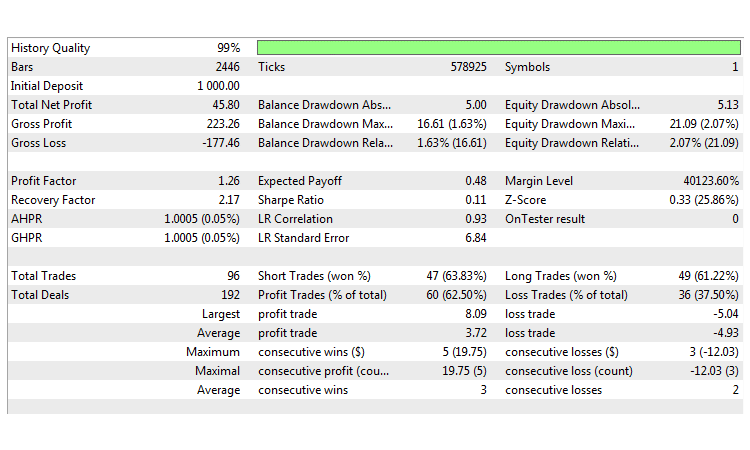```input int                  Inp_StopLoss=450;                            //止损(点数)

//--- 布林带参数
input int                  Inp_BBPeriod=14;                             //BB 周期
input double               Inp_deviation=2.0;                           //偏离
//--- 慢速均线参数
input int                  Inp_ma_period1=12;                           //慢速均线周期
input ENUM_MA_METHOD       Inp_ma_method1=MODE_SMMA;                    //慢速均线平滑方法
//--- 快速均线参数
input int                  Inp_ma_period2=2;                            //快速均线周期
input ENUM_MA_METHOD       Inp_ma_method2=MODE_LWMA;                    //快速均线平滑方法
```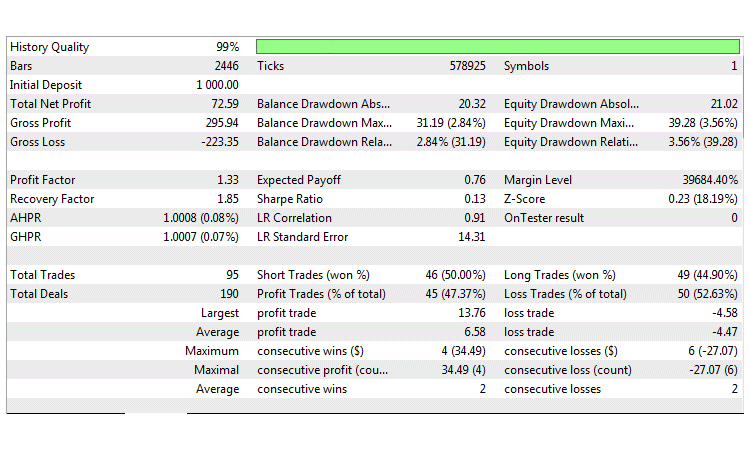```input int                  Inp_StopLoss=500;                            //止损(点数)

//--- WSO & WRO 通道参数
input int                  Inp_WsoWroPeriod=16;                         //Wso & Wro 通道周期
//--- 埃勒斯分形维度参数
input int                  Inp_FdiPeriod    =  18;                      //分形维度周期
input double               Inp_FdiThreshold =  1.4;                     //分形维度阈值
input ENUM_APPLIED_PRICE   Inp_Price        = PRICE_CLOSE;              //价格类型
```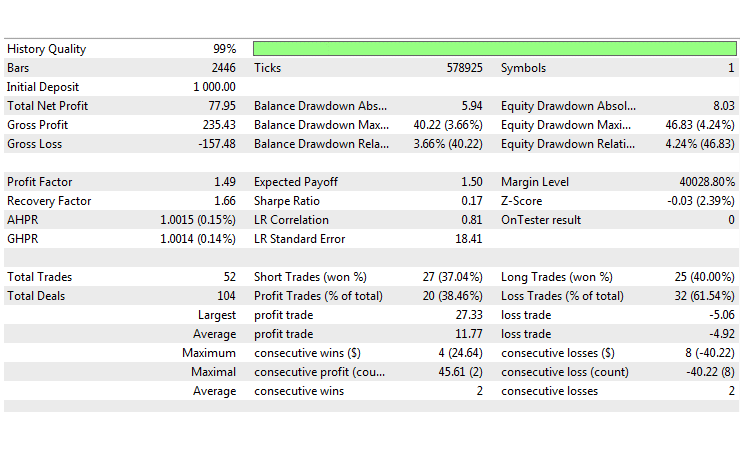```input int                  Inp_StopLoss=500;                            //止损(点数)

//--- Percentage_Crossover_Channel 参数
input double               Inp_Percent=26.0;                            //限定距离百分比
input ENUM_APPLIED_PRICE   Inp_Price=PRICE_CLOSE;                       //价格类型
//--- 趋势范围指标参数
input uint                 Inp_PeriodTR    =  14;                       //趋势范围指标周期
input ENUM_MA_METHOD       Inp_Method      =  MODE_EMA;                 //平滑方法
input double               Inp_Deviation   =  1.0;                      //偏离
```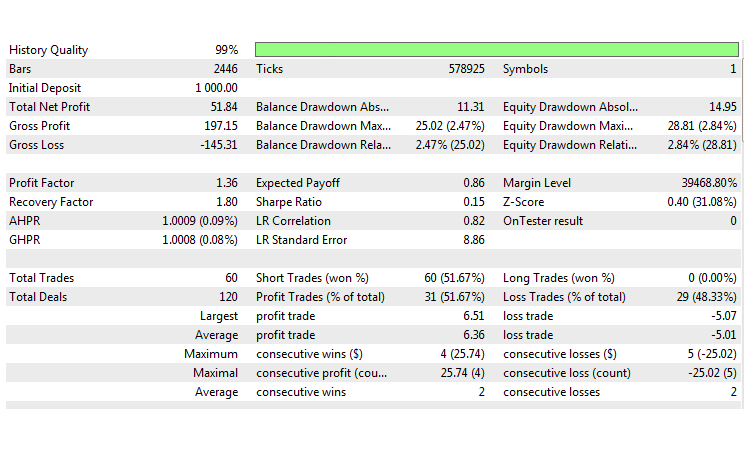```input int                  Inp_StopLoss=450;                            //止损(点数)

//--- 价格通道指标参数
input int                  Inp_ChannelPeriod=12;                        //周期
//--- RBVI 指标参数
input int                  Inp_RBVIPeriod=5;                            //RBVI 周期
input ENUM_APPLIED_VOLUME  Inp_VolumeType=VOLUME_TICK;                  //交易量
input double               Inp_level=40;                                //横盘级别
```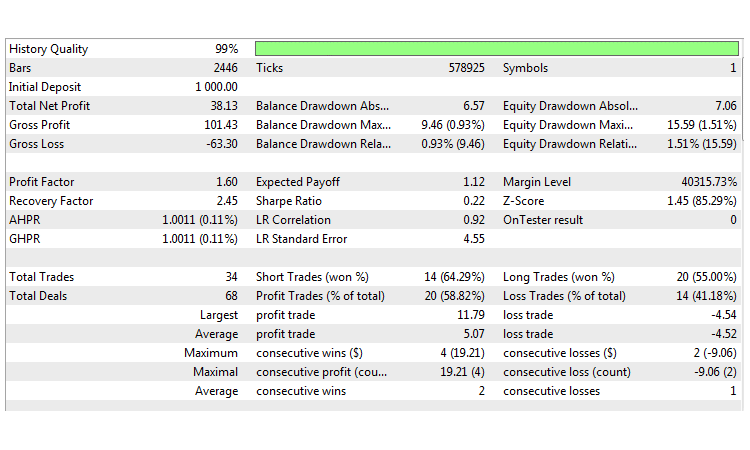```input int                  Inp_StopLoss=50;                             //止损(点数)
input int                  Inp_TakeProfit=50;                           //止盈(点数)

//--- WPR 指标参数
input int                  Inp_WPRPeriod=10;                            // WPR 周期
```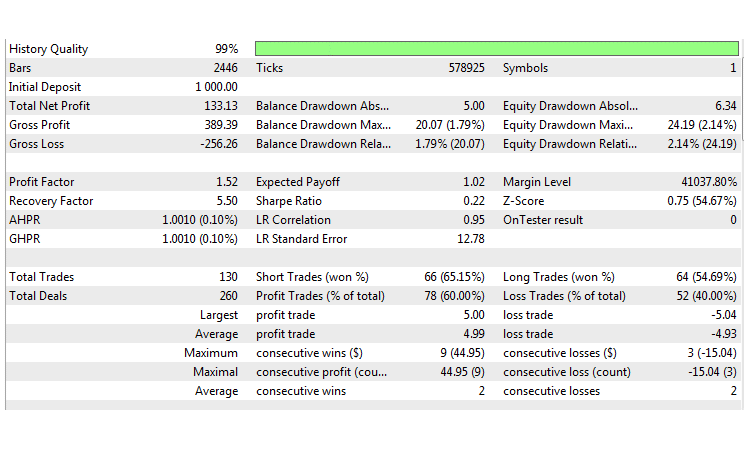```input int                     Inp_SmoothCenter      =  11;              // 平滑中心线的周期数字
input int                     Inp_SmoothDeviation   =  12;              // 平滑偏差的周期数字
input double                  Inp_F                 =  1.0;             // 作用于偏差的因子
input ENUM_APPLIED_PRICE      Inp_AppliedPrice      =  PRICE_CLOSE;     // 中心线应用价格:
input ENUM_MA_METHOD          Inp_MethodSmoothing   =  MODE_SMA;        // 中心线平滑方法
input ENUM_METHOD_VARIATION   Inp_MethodVariation   =  METHOD_HL;       // 变异方法
//--- 魔幻趋势指标参数
input uint                    Inp_PeriodCCI   =  60;                    // CCI 周期
input uint                    Inp_PeriodATR   =  5;                     // ATR 周期
```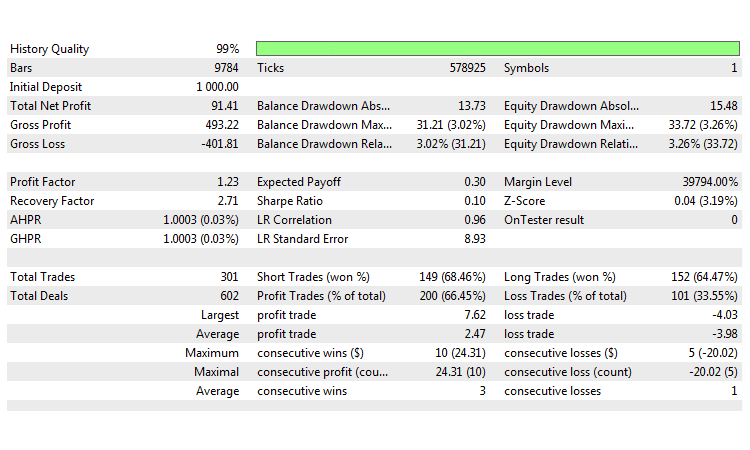```input int                  Inp_StopLoss=500;                            //止损(点数)

//--- 唐奇安通道参数
input int                  Inp_ChannelPeriod=12;                        //唐奇安周期
//--- 三位一体脉动指标参数
input int                  Inp_Period= 5;                               //指标周期
input int                  Inp_Level= 34;                               //平滑级别
input ENUM_MA_METHOD       Inp_Type=MODE_LWMA;                          //均化类型
input ENUM_APPLIED_PRICE   Inp_Price=PRICE_WEIGHTED;                    //价格类型
input ENUM_APPLIED_VOLUME  Inp_Volume=VOLUME_TICK;                      //成交量类型
```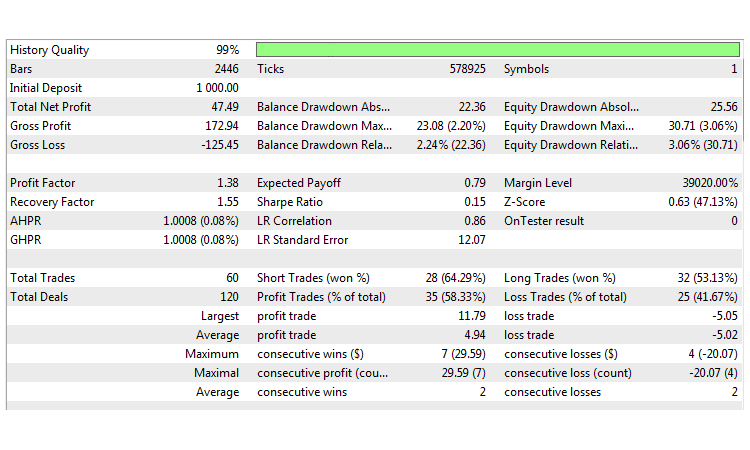```//--- ATR 通道参数
input ENUM_MA_METHOD       Inp_MA_Method=MODE_SMA;                      //均线平滑方法
input uint                 Inp_MA_Period=10;                            //均线周期
input uint                 Inp_ATR_Period=12;                           //ATR 周期
input double               Inp_Factor=1.5;                              //偏离数字
input ENUM_APPLIED_PRICE   Inp_IPC=PRICE_LOW;                           //价格类型
input int                  Inp_Shift=0;                                 //指标平移的柱线数
//--- CCI 色阶参数
input int                  Inp_CCI_ma_period = 14;                      // 均化周期
input double               Inp_CCI_LevelUP   = 90;                      // 向上级别
input double               Inp_CCI_LevelDOWN =-90;                      // 向下级别
```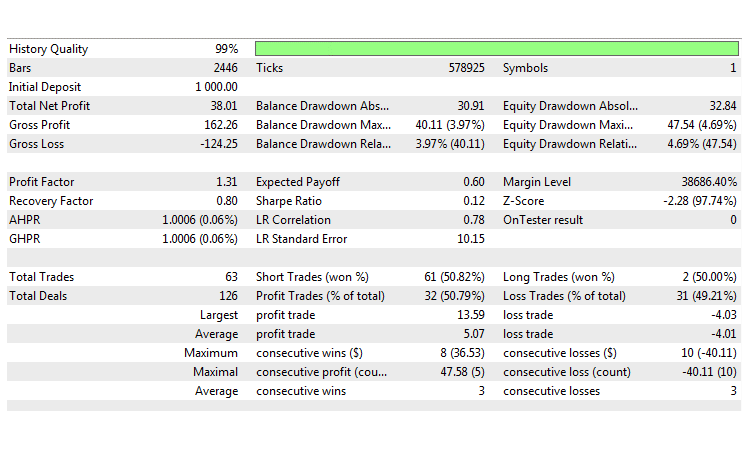```//--- RSI 直方图参数
input uint                 Inp_RSIPeriod=12;                            // 指标周期
input ENUM_APPLIED_PRICE   Inp_RSIPrice=PRICE_CLOSE;                    // 价格类型
input uint                 Inp_HighLevel=60;                            // 超买级别
input uint                 Inp_LowLevel=40;                             // 超卖级别
input int                  Inp_Shift=0;                                 // 指标平移的柱线数
//--- 横盘指标参数
input uint                 Inp_Smooth=10;                               // 平滑周期
input ENUM_MA_METHOD       Inp_ma_method=MODE_SMA;                      // 平滑类型
input ENUM_APPLIED_PRICE   Inp_applied_price=PRICE_CLOSE;               // 价格类型
input uint                 Inp_HLRef=100;
input int                  Inp_FShift=0;                                // 指标平移的柱线数
input uint                 Inp_ExtraHighLevel=70;                       // 最大趋势级别
input uint                 Inp_FHighLevel=50;                           // 强趋势级别
input uint                 Inp_FLowLevel=30;                            // 弱趋势级别
```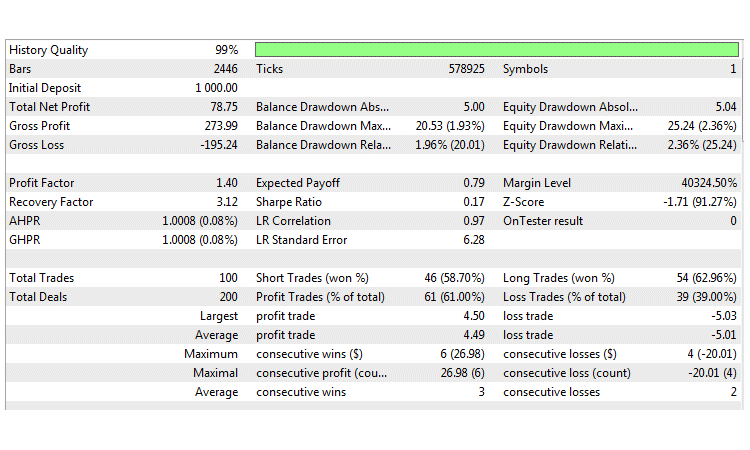### 研究成果

• 大多数策略都是基于通道内的交易，并包含信号过滤，因此它们的主要弱点是短线通道突破。
• 在非常低和非常高的时间帧内进行的测试均亏损，这分别由于入场条件过于频繁，以及选择性极端严苛。
• 在同一货币对和时间段的优化期间，所获回报没有明显的差别。 几乎所有策略都表现出类似的结果。

### 结束语

#
名称

1
Strategy_1.mq5

策略 #1. 附带基于 MFI 滤波器的轨道线指标
2
Strategy_2.mql5

策略 #2. 布林带加两条移动均线
3
Strategy_3.mq5

4
Strategy_4.mq5

策略 #4， 通道交叉百分比指标并基于趋势范围滤波器
5
Strategy_5.mq5 智能交易系统  策略 #5， 价格通道指标和基于 RBVI 的滤波器
6
Strategy_6.mq5

7 Strategy_7.mq5

策略 #7， 改编的 Keltner 通道和基于魔幻趋势的滤波器
8 Strategy_8.mq5 智能交易系统  策略 #8， 唐奇安 (Donchian) 通道，由三位一体脉动 (Trinity Impulse) 确认
9 Strategy_9.mq5 智能交易系统  策略 #9， ATR 通道指标和基于 CCI 色阶的滤波器
10 Strategy_10.mq5 智能交易系统  策略 #10， RSI 直方图和基于横盘指标的滤波器
13 wwc.mq5 指标  用在策略 #3
14 fdi.mq5 指标  用在策略 #3
15 pcc.mq5 指标  用在策略 #4
16 trend_range.mq5  指标  用在策略 #4
17 price_channel.mq5 指标  用在策略 #5
18 rbvi.mq5 指标  用在策略 #5
19 customizable _keltner.mq5 指标  用在策略 #7
20 magic_trend.mq5 指标  用在策略 #7
21 donchian_channel.mq5 指标  用在策略 #8
22 trinity_impulse.mq5 指标  用在策略 #8
23 atr_channel.mq5 指标   用在策略 #9
24 cci_color_levels.mq5 指标  用在策略 #9
25 rsi_histogram.mq5 指标  用在策略 #10
26 flat.mq5 指标  用在策略 #10

10Flat.zip (60.96 KB)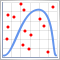蒙特卡洛方法在交易策略优化中的应用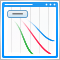改进面板：增加透明化、改变背景色以及继承于 CAppDialog/CWndClient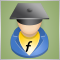在 EA 交易代码中实现指标的计算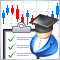如何创建订购交易机器人的需求规范